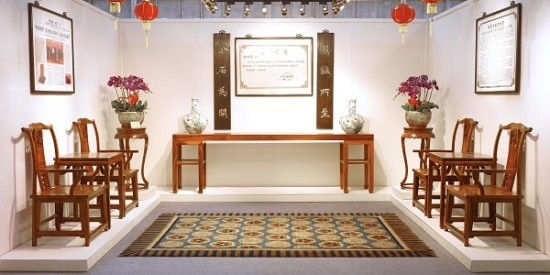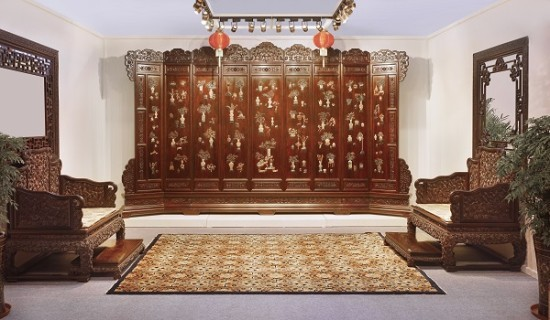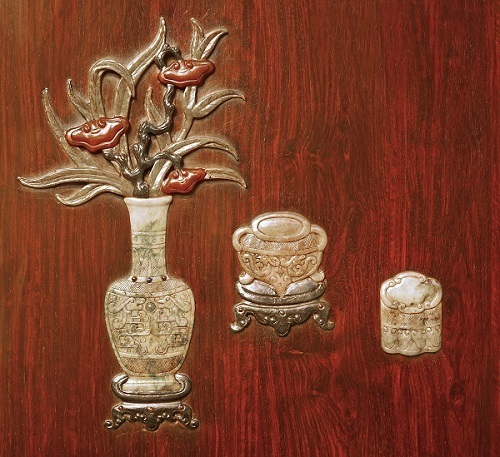|

# “岭南鲁班”伍炳亮创文博会特别金奖“十一连冠”

近日，第十三届中国(深圳)国际文化产业博览交易会“中国工艺美术文化创意奖”在深圳会展中心揭晓，经过激烈的角逐，在1963件参评作品里评选出12件特别金奖作品，广东独占7件，拔得头筹。其中，素有“岭南鲁班”之誉的中国传统家具设计大师、中国工美行业艺术大师、中国家具协会传统家具专业委员会主席伍炳亮创新设计的作品《清式檀香紫檀镶玉花卉百宝纹九屏风地座屏》以其用料豪奢大气，雕工细腻精美，榫接巧妙合理，镶嵌严谨考究，型制宏伟庄重，从众多参评作品中脱颖而出，摘得本奖项特别金奖。这是伍炳亮继“明式海南黄花梨透雕螭龙纹八扇大围屏”、“明式海南黄花梨大号月洞门架子床”等作品在连续十次蝉联该奖项之后，第十一次获此殊荣。深圳文博会是中国唯一的国家级、国际化、综合性的文化产业博览交易盛会，被誉为“中国文化产业第一展”，至今已连续成功举办十三届。文博会浓缩了中国文化精华，集中展示了中国最优秀的文化产品和文化成果，成为中国文化产业整体实力展示的舞台。其中，展会设立的“中国工艺美术文化创意奖”以追求原创、突出精品为主旨，是中国工艺美术设计界最高规格的评奖活动，而特别金奖的规格更是堪比国内工艺美术界的“奥斯卡”。该奖项的评选规则十分严格，其中要求特别金奖的获奖作品必须吻合时代需求，在继承传统的基础上予以发展与创新，并在相应的工艺美术类别中代表我国工艺美术设计制作的最高水准。伍炳亮地座屏作品此次荣获特别金奖，创下了“十一连冠”的佳绩，成为文博会上当之无愧的无冕之王。第十三届文博会伍氏兴隆展厅内景

清宫重器惊现文博会，艺压群雄斩获创意金奖在这次的文博会上，9号馆工艺美术展馆里的伍氏兴隆展厅在众多的展厅中显得格外引人瞩目，独具特色的岭南古典建筑样式，黑、白、灰三种简约而又明确的色调，构成了和谐古雅的主体外观，青砖白瓦的外墙装饰，以及门口“清风明韵、姚黄魏紫”的匾牌，顿时让人感到一股雅韵古风之气扑面而来。展馆内部由两个大厅和四个房间构成，伍炳亮按照清式宫廷紫檀系列、明式海南黄花梨书房系列、明式越南黄花梨卧室系列、清式老红酸枝客厅系列等，将精品力作进行了分类展示，整个展厅显得美轮美奂而又错落有致，在这一方天地里构筑起中国传统家具的艺术殿堂。伍炳亮这次在文博会上获得金奖的《清式檀香紫檀镶玉花卉百宝纹九屏风地座屏》，采用野生印度檀香紫檀老料精制而成，檀香紫檀在古代印度是圣树，因其木质坚硬，香气芬芳永恒，且百毒不侵，万古不朽，又能避邪，故称圣檀，人们常把其作为吉祥之物。檀香紫檀生长缓慢，有百年寸檀的说法；而且出材率很低，正所谓“十檀九空”，大凡紫檀原木多有空心，因此规格大的紫檀家具价格都会高得出奇。此屏风高达255公分，围长430公分，进深56公分，如此体量的檀香紫檀家具极为少见，仅从用材上便可看出其价值不菲。屏体共由九扇围屏组成，扇扇精雕细刻，处处玲珑剔透。首尾两扇斜向外展，中间七扇纵贯一气；全屏气象恢宏，巍然肃穆。其用材极尽奢华，加上出神入化的雕刻技艺，将皇家宫廷的豪华富贵气象烘托得淋漓尽致。屏帽锼成如意云形，其上浮雕草叶花纹，雕工精微，布局合理。每扇屏心采用百宝嵌工艺，均以各色翡翠、玉石为饰，镶嵌出各种鲜花异卉、文玩博古，其色五彩斑斓，其韵生动雅致。屏心所用百宝嵌工艺以是金银、宝石、珍珠、珊瑚、碧玉、翡翠、水晶、玛瑙、玳瑁、车渠、青金、绿松、螺钿、象牙、蜜蜡、沉香等材料，根据画面需要而镶嵌在器物表面的装饰工艺的总称，以用料奇珍、工艺复杂而闻名。屏心上下攒框刷槽装绦环板，其上亦雕以草叶花纹，丰腴饱满，精美雅致。屏身下承须弥座，气势雄浑，底部则以云纹足落地，委婉承重，匠心独运。九扇围屏以榫卯相联，合围而立，肃穆庄严。在清式宫廷家具的范畴中，这种大型屏风多和宝座一起配套使用，规格等级很高，多作为礼器使用，是帝后级人物的专享，其他人不得僭制使用，因此在选材用料和造型权衡等各方面都极为考究。这件地座屏作品用料豪奢大气，雕工细腻精美，榫接巧妙合理，镶嵌严谨考究，型制宏伟庄重，品韵富丽华美；既传承了清代宫廷家具制作精华，深追清宫御制之精要，同时又融入了个人的思想情感和创意设计；秉承清代宫廷家具之精髓而又别开生面，恢宏大气而又极尽工艺之美，实为借古开今、传昭后世的精品重器，令到场的观众和专家赞不绝口，毫无争议地斩获此次文博会“中国工艺美术文化创意奖”的特别金奖。清式 檀香紫檀镶玉花卉百宝纹九屏风地座屏(伍炳亮作品)

续接前贤超出同侪，复现绝艺借古开今从上世纪80年代初以来，伍炳亮从事传统家具的设计制作与购藏、研究已达三十多年之久，在传统家具的制作及造型审美的认识方面积累了丰富的经验，设计制作出数千款家具作品，涵盖了传统家具的各个类别，大体以明式黄花梨及清式紫檀为重要代表，糅合了明清家具艺术的各类风格。在中国家具史上，明式家具简练空灵、古雅精丽，而清式宫廷家具则繁纹重饰，富丽华贵，成为中国家具史上的两座高峰。清朝设造办处，从全国选调最好的工匠进京，成为宫廷御用匠人，精研出了雕、嵌、描、堆等多种技法，工艺繁杂，形式多变，使清式宫廷家具成为集历代传统家具工艺之大成者。可惜的是，随着时代的变迁，这些工艺瑰宝传至今日很多已经濒临灭绝，这造成当代清式仿古家具多风格趋同，程式化严重，常让人生出“千器一面”的喟叹。令人欣慰的是，伍炳亮的清式家具作品不落此的程式化俗套，他穷三十余年之力，深研清式宫廷家具的工艺技法，不仅完整系统的继承了传统工艺精髓，款型丰富雅正、雕工细致入微、刀法风格多变，且能在曲尽其妙之间别出心裁，借古开今。以这次荣获金奖的《清式檀香紫檀镶玉花卉百宝纹九屏风地座屏》为例，此屏风所用的镶嵌工艺又名“百宝嵌”，史称“周制”、“周嵌”，以其为晚明周姓者创制而得名。据清代钱泳的《履园丛话》记载：“其法以金、银、宝石、珍珠、青金、绿松、螺钿、象牙、蜜蜡、沉香为之，雕成山水、人物、树木、楼台、花卉、翎毛，嵌于檀、梨、漆器之上。五色陆离，难以形容，真古来未有之奇玩也。”史传乾隆皇帝既喜爱玉器，也珍爱紫檀，为此宫廷造办处特地制作了不少紫檀嵌玉家具，这件地座屏便是在乾隆御制的原款基础上改制而成。由此可见，精选珍惜材料，用以嵌饰并构图成趣，构成了“百宝嵌”精彩纷呈、美轮美奂的艺术审美特征。遗憾的是这项传统工艺瑰宝传至今日，已经罕有人可以完整掌握，甚至一度濒临失传。而伍炳亮深受岭南广作用料不吝其奢，做工不厌其精，工艺种类齐备繁多的影响，故而在传承镶嵌工艺手法方面，具有近水楼台先得月的天然优势。经过三十余年的厚积薄发，伍炳亮已经将传统家具的雕饰手法运用得炉火纯青，就中便包括百宝嵌工艺。从《清式檀香紫檀镶玉花卉百宝纹九屏风地座屏》来看，伍炳亮对百宝嵌工艺的掌握已经非常纯熟，其雕嵌雅正、型制规整，深得传统工艺的精髓。尤其难得是，该屏在复现出清式宫廷家具华贵庄重的神韵的同时，更有清雅脱俗之姿，而无清宫御制甜俗软媚之弊。清式 檀香紫檀镶玉花卉百宝纹九屏风地座屏局部(伍炳亮作品)

获奖秘诀：“大国工匠”超越历史，继承传统贵在创新从这件《清式檀香紫檀镶玉花卉百宝纹九屏风地座屏》可以看出，伍炳亮对传统工艺的继承，不仅可以接续前贤，甚至能超越历史水平，表现出强烈的个性特征和时代精神。在经历了三十余年制器生涯的磨练之后，对于传统家具的设计制作，伍炳亮更强调个人对传统家具文化本身的理解和演绎，来真正树立属于时代、属于自己的风格。伍炳亮告诉记者：“传统家具的历史是活的，不是死的，它生动地呈现在一件件老家具款型身上。但款型则有好坏优劣之分，需要我们凭借自己的理解去区别和领悟。所以设计制作传统家具，既不能照本宣科、一比一地去复制，也不能生搬硬套地胡乱臆造，或是急功近利、刻意地标新立异。”这件地座屏就是伍炳亮这种传统家具设计理念具体而微的体现，此屏风本身的款型非常经典，乃是仿照了故宫博物院的旧藏原款《清乾隆 紫檀木边嵌玉石花卉图围屏》，但规格尺寸比原款更大。从选材、工艺及表现内容来看，这件作品虽是仿作，但并不拘泥于古人，而是加入了自己的理解和创意。故宫原款屏风是紫檀的外框，杂木制成的屏心，屏上用米黄色漆地，上面再嵌玉石花卉，并加乾隆御题诗。这件屏风新作则通体选用紫檀，原作屏心的四时花卉被各式插花、文玩、博古所取代，乾隆御诗亦被剔除，其构思精巧，嵌饰精湛，与原作的皇家富贵、艳俗之气相比，更显古雅隽永之书卷气息，使其更具亲和力，也更符合当代人的审美理想。著名作家、传统家具收藏家海岩评价伍炳亮的艺术成就时说：“中国明清两代硬木家具分别代表了士大夫和皇室贵族的审美趣味，两者风格虽然简繁各异，趣味不同，但都是精英文化和审美品味结合精绝工艺与珍贵材质，融贯而成的艺术形式，在世界艺术领域独具特色。而当今社会，整体心态偏向浮躁和急功近利，市场上的仿古家具也难见上等精品，能够对传统家具深入研究并身体力行去全面制作继承的高手实不多见。伍炳亮先生三十年如一日孜孜不倦地投身家具设计与制作，其家具作品既融汇了传统家具各大风格流派之所长，又前后贯穿、表现了在不同时期的制器风貌。”其实，从这件《清式檀香紫檀镶玉花卉百宝纹九屏风地座屏》来看，伍炳亮不仅是一个全面继承传统的高手，更将明清家具两种风格融会贯通，把皇家贵胄和文人士大夫的审美趣味熔冶于一炉，并注入当代人的审美理想，打破了时代与工艺的壁垒，从而成为新时代当之无愧的“大国工匠”。这也许就是伍炳亮能够在历届文博会上艺压群雄，创下特别金奖“十一连冠”的秘诀吧！

`声明：本文由入驻焦点开放平台的作者撰写，除焦点官方账号外，观点仅代表作者本人，不代表焦点立场错误信息举报电话： 400-099-0099，邮箱：jubao@vip.sohu.com，或点此进行意见反馈，或点此进行举报投诉。`A B C D E F G H J K L M N P Q R S T W X Y Z
A - B - C - D - E
• A
• 鞍山
• 安庆
• 安阳
• 安顺
• 安康
• 澳门
• B
• 北京
• 保定
• 包头
• 巴彦淖尔
• 本溪
• 蚌埠
• 亳州
• 滨州
• 北海
• 百色
• 巴中
• 毕节
• 保山
• 宝鸡
• 白银
• 巴州
• C
• 承德
• 沧州
• 长治
• 赤峰
• 朝阳
• 长春
• 常州
• 滁州
• 池州
• 长沙
• 常德
• 郴州
• 潮州
• 崇左
• 重庆
• 成都
• 楚雄
• 昌都
• 慈溪
• 常熟
• D
• 大同
• 大连
• 丹东
• 大庆
• 东营
• 德州
• 东莞
• 德阳
• 达州
• 大理
• 德宏
• 定西
• 儋州
• 东平
• E
• 鄂尔多斯
• 鄂州
• 恩施
F - G - H - I - J
• F
• 抚顺
• 阜新
• 阜阳
• 福州
• 抚州
• 佛山
• 防城港
• G
• 赣州
• 广州
• 桂林
• 贵港
• 广元
• 广安
• 贵阳
• 固原
• H
• 邯郸
• 衡水
• 呼和浩特
• 呼伦贝尔
• 葫芦岛
• 哈尔滨
• 黑河
• 淮安
• 杭州
• 湖州
• 合肥
• 淮南
• 淮北
• 黄山
• 菏泽
• 鹤壁
• 黄石
• 黄冈
• 衡阳
• 怀化
• 惠州
• 河源
• 贺州
• 河池
• 海口
• 红河
• 汉中
• 海东
• 怀来
• I
• J
• 晋中
• 锦州
• 吉林
• 鸡西
• 佳木斯
• 嘉兴
• 金华
• 景德镇
• 九江
• 吉安
• 济南
• 济宁
• 焦作
• 荆门
• 荆州
• 江门
• 揭阳
• 金昌
• 酒泉
• 嘉峪关
K - L - M - N - P
• K
• 开封
• 昆明
• 昆山
• L
• 廊坊
• 临汾
• 辽阳
• 连云港
• 丽水
• 六安
• 龙岩
• 莱芜
• 临沂
• 聊城
• 洛阳
• 漯河
• 娄底
• 柳州
• 来宾
• 泸州
• 乐山
• 六盘水
• 丽江
• 临沧
• 拉萨
• 林芝
• 兰州
• 陇南
• M
• 牡丹江
• 马鞍山
• 茂名
• 梅州
• 绵阳
• 眉山
• N
• 南京
• 南通
• 宁波
• 南平
• 宁德
• 南昌
• 南阳
• 南宁
• 内江
• 南充
• P
• 盘锦
• 莆田
• 平顶山
• 濮阳
• 攀枝花
• 普洱
• 平凉
Q - R - S - T - W
• Q
• 秦皇岛
• 齐齐哈尔
• 衢州
• 泉州
• 青岛
• 清远
• 钦州
• 黔南
• 曲靖
• 庆阳
• R
• 日照
• 日喀则
• S
• 石家庄
• 沈阳
• 双鸭山
• 绥化
• 上海
• 苏州
• 宿迁
• 绍兴
• 宿州
• 三明
• 上饶
• 三门峡
• 商丘
• 十堰
• 随州
• 邵阳
• 韶关
• 深圳
• 汕头
• 汕尾
• 三亚
• 三沙
• 遂宁
• 山南
• 商洛
• 石嘴山
• T
• 天津
• 唐山
• 太原
• 通辽
• 铁岭
• 泰州
• 台州
• 铜陵
• 泰安
• 铜仁
• 铜川
• 天水
• 天门
• W
• 乌海
• 乌兰察布
• 无锡
• 温州
• 芜湖
• 潍坊
• 威海
• 武汉
• 梧州
• 渭南
• 武威
• 吴忠
• 乌鲁木齐
X - Y - Z
• X
• 邢台
• 徐州
• 宣城
• 厦门
• 新乡
• 许昌
• 信阳
• 襄阳
• 孝感
• 咸宁
• 湘潭
• 湘西
• 西双版纳
• 西安
• 咸阳
• 西宁
• 仙桃
• 西昌
• Y
• 运城
• 营口
• 盐城
• 扬州
• 鹰潭
• 宜春
• 烟台
• 宜昌
• 岳阳
• 益阳
• 永州
• 阳江
• 云浮
• 玉林
• 宜宾
• 雅安
• 玉溪
• 延安
• 榆林
• 银川
• Z
• 张家口
• 镇江
• 舟山
• 漳州
• 淄博
• 枣庄
• 郑州
• 周口
• 驻马店
• 株洲
• 张家界
• 珠海
• 湛江
• 肇庆
• 中山
• 自贡
• 资阳
• 遵义
• 昭通
• 张掖
• 中卫

1室1厅1厨1卫1阳台

1
2
3
4
5

0
1
2

1

1

0
1
2
3报名成功，资料已提交审核A B C D E F G H J K L M N P Q R S T W X Y Z
A - B - C - D - E
• A
• 鞍山
• 安庆
• 安阳
• 安顺
• 安康
• 澳门
• B
• 北京
• 保定
• 包头
• 巴彦淖尔
• 本溪
• 蚌埠
• 亳州
• 滨州
• 北海
• 百色
• 巴中
• 毕节
• 保山
• 宝鸡
• 白银
• 巴州
• C
• 承德
• 沧州
• 长治
• 赤峰
• 朝阳
• 长春
• 常州
• 滁州
• 池州
• 长沙
• 常德
• 郴州
• 潮州
• 崇左
• 重庆
• 成都
• 楚雄
• 昌都
• 慈溪
• 常熟
• D
• 大同
• 大连
• 丹东
• 大庆
• 东营
• 德州
• 东莞
• 德阳
• 达州
• 大理
• 德宏
• 定西
• 儋州
• 东平
• E
• 鄂尔多斯
• 鄂州
• 恩施
F - G - H - I - J
• F
• 抚顺
• 阜新
• 阜阳
• 福州
• 抚州
• 佛山
• 防城港
• G
• 赣州
• 广州
• 桂林
• 贵港
• 广元
• 广安
• 贵阳
• 固原
• H
• 邯郸
• 衡水
• 呼和浩特
• 呼伦贝尔
• 葫芦岛
• 哈尔滨
• 黑河
• 淮安
• 杭州
• 湖州
• 合肥
• 淮南
• 淮北
• 黄山
• 菏泽
• 鹤壁
• 黄石
• 黄冈
• 衡阳
• 怀化
• 惠州
• 河源
• 贺州
• 河池
• 海口
• 红河
• 汉中
• 海东
• 怀来
• I
• J
• 晋中
• 锦州
• 吉林
• 鸡西
• 佳木斯
• 嘉兴
• 金华
• 景德镇
• 九江
• 吉安
• 济南
• 济宁
• 焦作
• 荆门
• 荆州
• 江门
• 揭阳
• 金昌
• 酒泉
• 嘉峪关
K - L - M - N - P
• K
• 开封
• 昆明
• 昆山
• L
• 廊坊
• 临汾
• 辽阳
• 连云港
• 丽水
• 六安
• 龙岩
• 莱芜
• 临沂
• 聊城
• 洛阳
• 漯河
• 娄底
• 柳州
• 来宾
• 泸州
• 乐山
• 六盘水
• 丽江
• 临沧
• 拉萨
• 林芝
• 兰州
• 陇南
• M
• 牡丹江
• 马鞍山
• 茂名
• 梅州
• 绵阳
• 眉山
• N
• 南京
• 南通
• 宁波
• 南平
• 宁德
• 南昌
• 南阳
• 南宁
• 内江
• 南充
• P
• 盘锦
• 莆田
• 平顶山
• 濮阳
• 攀枝花
• 普洱
• 平凉
Q - R - S - T - W
• Q
• 秦皇岛
• 齐齐哈尔
• 衢州
• 泉州
• 青岛
• 清远
• 钦州
• 黔南
• 曲靖
• 庆阳
• R
• 日照
• 日喀则
• S
• 石家庄
• 沈阳
• 双鸭山
• 绥化
• 上海
• 苏州
• 宿迁
• 绍兴
• 宿州
• 三明
• 上饶
• 三门峡
• 商丘
• 十堰
• 随州
• 邵阳
• 韶关
• 深圳
• 汕头
• 汕尾
• 三亚
• 三沙
• 遂宁
• 山南
• 商洛
• 石嘴山
• T
• 天津
• 唐山
• 太原
• 通辽
• 铁岭
• 泰州
• 台州
• 铜陵
• 泰安
• 铜仁
• 铜川
• 天水
• 天门
• W
• 乌海
• 乌兰察布
• 无锡
• 温州
• 芜湖
• 潍坊
• 威海
• 武汉
• 梧州
• 渭南
• 武威
• 吴忠
• 乌鲁木齐
X - Y - Z
• X
• 邢台
• 徐州
• 宣城
• 厦门
• 新乡
• 许昌
• 信阳
• 襄阳
• 孝感
• 咸宁
• 湘潭
• 湘西
• 西双版纳
• 西安
• 咸阳
• 西宁
• 仙桃
• 西昌
• Y
• 运城
• 营口
• 盐城
• 扬州
• 鹰潭
• 宜春
• 烟台
• 宜昌
• 岳阳
• 益阳
• 永州
• 阳江
• 云浮
• 玉林
• 宜宾
• 雅安
• 玉溪
• 延安
• 榆林
• 银川
• Z
• 张家口
• 镇江
• 舟山
• 漳州
• 淄博
• 枣庄
• 郑州
• 周口
• 驻马店
• 株洲
• 张家界
• 珠海
• 湛江
• 肇庆
• 中山
• 自贡
• 资阳
• 遵义
• 昭通
• 张掖
• 中卫• 手机• 分享
• 设计
免费设计
• 计算器
装修计算器
• 入驻
合作入驻
• 联系
联系我们
• 置顶
返回顶部